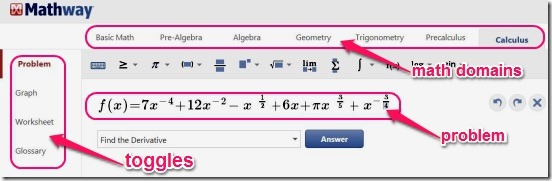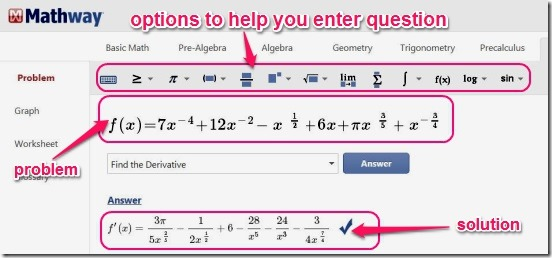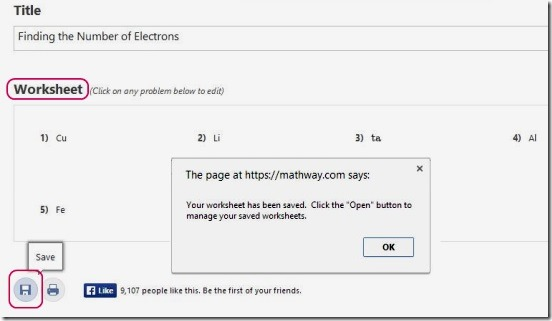Editor Ratings:
User Ratings:
[Total: 0 Average: 0]

Mathway is a free online math problem solver that solves basic and advanced math problems. It can solve basic math, pre-algebra, algebra, calculus, pre-calculus, geometry, statistics, liner math, algebra, and chemistry problems. You can quickly find the correct answers to the complex questions, and then save them in the website. Besides solving math questions in seconds, you can also solve and plot graphs, create and save math worksheets, and learn more about mathematics by using the Glossary section of the website.This is a pretty good website, that can help students of all levels in learning math and, generate interest in math. The website also shows a step by step explanation, showing each single step for solving the problem. But, this feature comes with the paid version. Still, the features available in free sign-up, are good enough to get the work done.## Using this online math problem solver to solve complex math problems:

This website is pretty helpful to students in learning and solving complex math problems. The range of different Math Domains (statistics, calculus, geometry, trigonometry etc.), that this math based website covers is pretty commendable. Because of this wide range, you can quickly find the right answer of almost any math problem. All you have to do is simply type the math problem in the text box and click on Answer button. The website shows you the solution within seconds.

The interface is well laid out, making it easy to work. All the different math domains are present at the top, and the major actions that you’ll do in the website are at the left in the form of toggle buttons. These include: Problem, Graph, Worksheet, and Glossary.It’s very easy to quickly jump between different math domains. Simply click on the desired domain in the interface, and select the desired toggle (for example Problem to simply solve a problem) and then enter the desired problem.

To make it easy for you to enter the desired question (for example the trigonometric problems may consist Pi, Theta, Euler’s number, base 10 logarithm, base N logarithm etc.), the website also gives you option to enter the special symbols in the problem. The moment you click on a math domain, it automatically shows the symbols and options respective to the selected domain. This is a pretty good thing about the website.

Same is the process for entering in the graph plotting problems. The website automatically finds the solution and plots them on graph in a very clear way. This graph can be zoomed in/out by using center mouse wheel.One good thing about this website is you can easily and quickly create math worksheets. The website has pre-built options to set the worksheet specifications like: topic, number of questions, columns, and blank row. Using these you can create a math questionnaire worksheets. After creating a worksheet you can save them in the website. You can save not just the worksheets but each single problem that you solve in the website. There’s a save button at the bottom of the page.And to retrieve the saved problems and worksheet, simply click on the Folder button in the interface.## Key features of Mathway:

• Good online math problem solver website.
• Sign-up free and use limited but effective services.
• Solve algebra, trigonometry, calculus, statistics, geometry, chemistry etc. problems in second.
• Plot graph.
• Create math worksheets.
• Save problems and worksheets in website.
• Use Glossary to learn and understand math terms and concepts.

Also check out this good website, that can help children in learning maths: Math Live.

## Conclusion:

Mathway is a good website to serve as an online math problem solver. It’s simple, fast, and very much handy for the students, and can help them with their studies. Though, the free sign-up services does lack the option to see the steps for solving problem. Still, it pretty much manages to get the basic work done.

You might also like checking out this good website that lets you create online math worksheets and share, publish them.